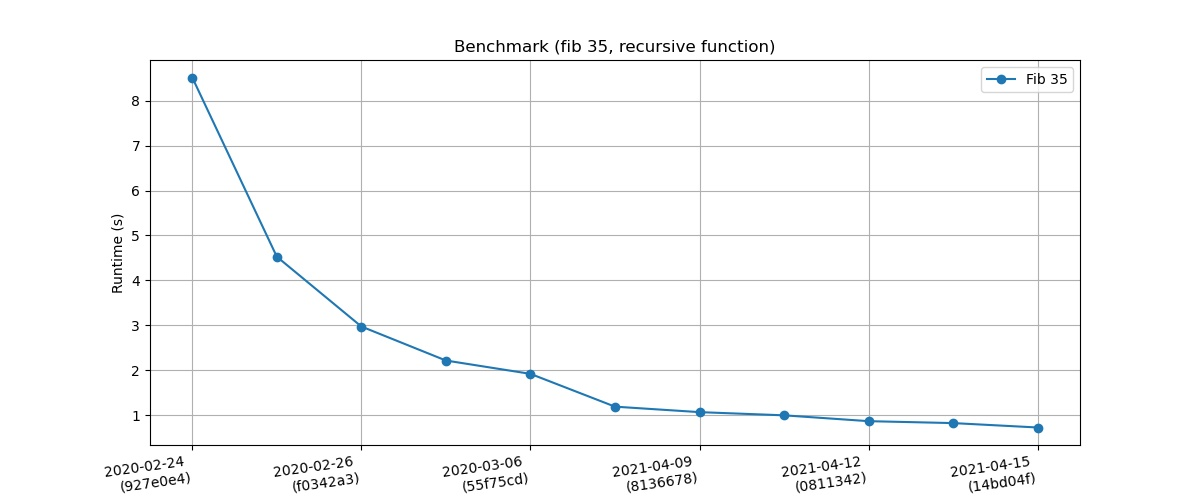## ~dvko/pepper-lang

Bytecode compiler and virtual machine for the Pepper toy programming language, hosted in C.
55e1f3d6 — Danny van Kooten 1 year, 8 months ago
`simplify ownership of instructions`
e48effac — Danny van Kooten 1 year, 8 months ago
`fix build, add more tests for str_split`
14de4a68 — Danny van Kooten 1 year, 8 months ago
`refactor GC into separate files, store gc meta on heap objects directly`

master
browse  log

### clone

https://git.sr.ht/~dvko/pepper-lang
git@git.sr.ht:~dvko/pepper-lang

You can also use your local clone with git send-email.

## #Pepper Programming LanguagePepper is an interpreted programming toy language that I wrote with a focus on simplicity and verbosity. It is dynamically typed and comes with built-in garbage collection. At the moment, it's basically a weird hybrid between C, JavaScript and Python.

It's also quite fast for an interpreted language that does not (yet) use JIT compilation.

Pepper is implemented in C11 with no external dependencies. It would have been ANSI standard if it weren't for the use of computed goto's in the VM main loop to improve CPU branch prediction.

#### #Syntax example

```// Variable declarations
let a = 5;
let b = 25;

// Reassign (previously declared) variables
a = a * 5;

// For loops
for (let i = 0; i < 10; i++) {
// i = 0 .. 9
}

// While loops
while (b > a) {
b = b - 5;
break;
}

// If statements
if (b == a || a == b) {
print("b equals a!");
}

// Strings
let c = "Hello world";
c = str_split(c, " ");
type(c); // "ARRAY"
len(c); // 2

// Arrays
let d = [5, true, "Pepper"];
d; // 5
array_push(d, 10);
array_pop(d); // 10

// Slices
let s = d[0:2]; // [5, true]

// Functions
let fibonacci = fn(x) {
if (x < 2) {
return x;
}

return fibonacci(x - 1) + fibonacci(x - 2);
}

// Built-in functions
print("35th fibonacci number is: ", fibonacci(35));
print("Type of c = ", type(c));
print("Length of c = ", len(c));
print("Integer value of true = ", int(true));
file_get_contents("file.txt");
```

More examples can be found in the examples directory.

#### #Usage

Build Pepper interpreter (and REPL)

``````make
``````

Launch the REPL

``````bin/pepper
``````

Interpret a Pepper script:

``````bin/pepper examples/arithmetic.pr
``````

Build & run tests

``````make check
``````

Install Pepper in your system's binaries

``````sudo make install
``````

#### #Benchmarks

A benchmark to calculate the 35th fibonacci number using a recursive function is run on every commit through this Github action workflow.For fun, I ran the same algorithm expressed in some other interpreted languages on the same hardware (my laptop). This is how Pepper compares:

Language fib-35 string-concat-count selection-sort
Pepper 0.720 0.024 0.349
Lua 5.4 0.830 0.030 0.156
Node 15 0.210 0.058 0.071
PHP 8.0 0.480 0.036 0.595
PHP 5.6 2.720
Pypy 7.3 0.240 0.154 0.192
Python 3.9 1.910 0.060 1.009
Ruby 3.0 0.800 0.102 0.877